Join the forum, it's quick and easy

Would you like to react to this message? Create an account in a few clicks or log in to continue.

# [已解決]關於Lisp程式在08版和10版上執行的問題

2 posters##[已解決]關於Lisp程式在08版和10版上執行的問題

(chku drawtime "drawtime")
(setq oce (getvar "cmdecho") ;store current cmdecho value
oldly (getvar "clayer") ;store current layer
oldblp(getvar "blipmode") ;store current blipmode value
#tp 0 ;box type
)
(setq oer *error* ;store AutoLisp error routine
*error* seterr) ;temporarily replace it
(setvar "cmdecho" 0)
(setvar "blipmode" 0)
(setvar "attdia" 0)
(initget (+ 1 2 4)) ;no null input, negative or zero values
(setq b (*(/ (getint "\n繪圖比例: ") 100.)
_cacdrunit
)
file(open (getpath "setup" 2) "r")
cx 5);defun loop test value
(while (= cx 5)
(prompt (strcat "\n目前圖框類別為 < " (itoa #tp) " >..."))
(initget 5 "T")
(setq cx (getint "\n類別(T)/A(0)平/A(1)平/A(2)平/A(3)平/A0直(6)/A1直(7)/A2直(8)/A3直(9): "))
(while (= cx "T")
(if(setq x0(getint(strcat "\n使用圖框類別 <"(itoa #tp)">: ")))(setq #tp x0))
(prompt (strcat "\n目前圖框類別為 < " (itoa #tp) " >"))
(initget 5 "T")
(setq cx(getint "\n類別(T)/A(0)平/A(1)平/A(2)平/A(3)平/A0直(6)/A1直(7)/A2直(8)/A3直(9): "))
)
;defun x0 & y0 to decide view block x,y scale, x1,y1 for limits
(if (< cx 4)
(progn
(repeat (1+ (* 2 cx)) (setq h0 (atof (read-line file))))
)
(and (> cx 5) (< cx 10)
(repeat (+ 1 (* 2 (- 9 cx))) (setq h0 (atof (read-line file))))
)
)
(cond
((and (>= cx 0)(< cx 4))
(setq x0 (* h0 b) y0 (* v0 b) x1 (* x0 1.05) y1 (* y0 1.05))
)
((and (> cx 5)(< cx 10))
(setq x0 (* v0 b) y0 (* h0 b) x1 (* x0 1.05) y1 (* y0 1.05))
)
(setq cx 5)
)
);end loop of while
(setvar "USERI2" (1+ cx))
(close file)
(setq g (/ (+ 10 (atoi (rtos x1))) 20)) ;settting grid distance in g
(if (< g 1) ;if grid distance small than 1
(or
(and (> x1 15) (setq g 1))
(and (> x1 8) (setq g 0.5))
(and (> x1 4) (setq g 0.25))
(and (> x1 1.5) (setq g 0.1))
(and (> x1 0.8) (setq g 0.25))
(setq g 0.2)
)
) ;end if
(if (> cx 5)
(setq p (list 0 y0))
(setq p '(0 0))
)
(command "_-layer" "s" "0" "")
(if (= cx 10)
(setup_boxi p b cx #tp)
)
(setvar "LTSCALE" b)
(command "_limits" "0,0" (list x1 y1))
(setq p (/ b _cacdrunit)
x1(tblnext "DIMSTYLE" 0)
x0(getvar "DIMSTYLE")
)
(while x1
(setq y1(cdr(assoc 2 x1)))
(command "_dim" "_restore" y1 "_dimscale" p "_save" y1 "_y" "_exit")
(setq x1(tblnext "DIMSTYLE"))
)
(command "_dim" "_restore" x0 "_exit"
"_'zoom" "_a" "_-layer" "_s" oldly "" "_view" "_s" "_all")
(setvar "textsize" (* b 0.7)) ;setting text hight
(setvar "snapunit" (list g g)) ;settting grid distance
(setvar "cmdecho" oce) ;restore previous value of cmdecho
(setvar "blipmode" oldblp) ;restore previous blipmode value
(setq *error* oer ;restore previous error handler
seterr nil
oce (if (> _cacdrunit 10) "公分" "公尺")
)
(prompt (strcat "\n\n規劃:\t單位: " oce ", 比例: 1/" (rtos(/(* 100 b)_cacdrunit)) ", 格點間距: " (rtos g) oce) )
(princ)

▼在08版可以正常叫出圖框▼在10版就會出現" ** 需要重生，無法透通。"ss_andy_ss 在 2012-12-21, 17:40 作了第 1 次修改ss_andy_ss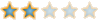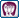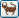##回復： [已解決]關於Lisp程式在08版和10版上執行的問題

(command "_dim" "_restore" x0 "_exit"
"_zoom" "_a" "_-layer" "_s" oldly "" "_view" "_s" "_all")pizg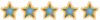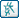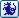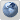##回復： [已解決]關於Lisp程式在08版和10版上執行的問題ss_andy_ss##回復： [已解決]關於Lisp程式在08版和10版上執行的問題

pizg 在 2012-12-27, 16:36 作了第 1 次修改pizg##回復： [已解決]關於Lisp程式在08版和10版上執行的問題ss_andy_ss